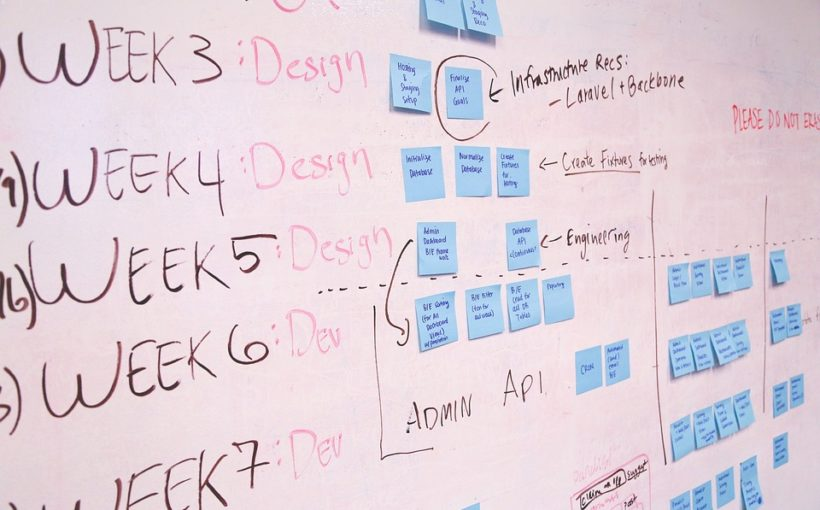# DDMRP : Buffer value calculation and dynamic adjustmentsPosted by

After the first posts regarding the DDMRP and Inventory Positioning and buffer level calculation, let’s see how we can automatize the buffer calculations and adjust them afterwards.

From the Item Coverage, let’s tick the Buffer values over time (this will blank defaulted the Min, Max and Reorder point) and move on.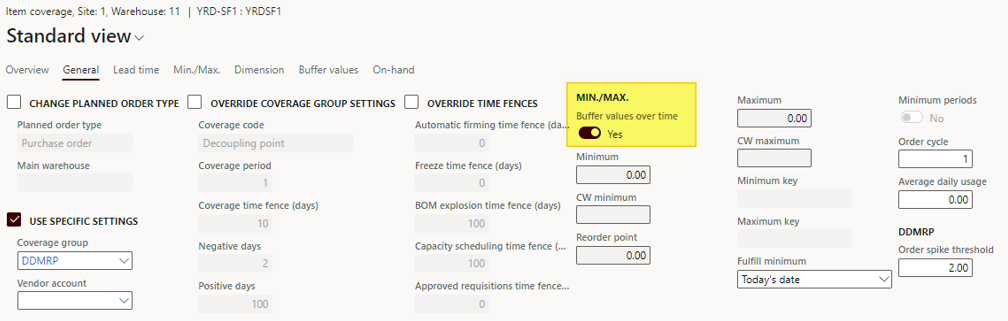We will let the system calculate the buffer values. Under the Buffer values tab, we can add time periods.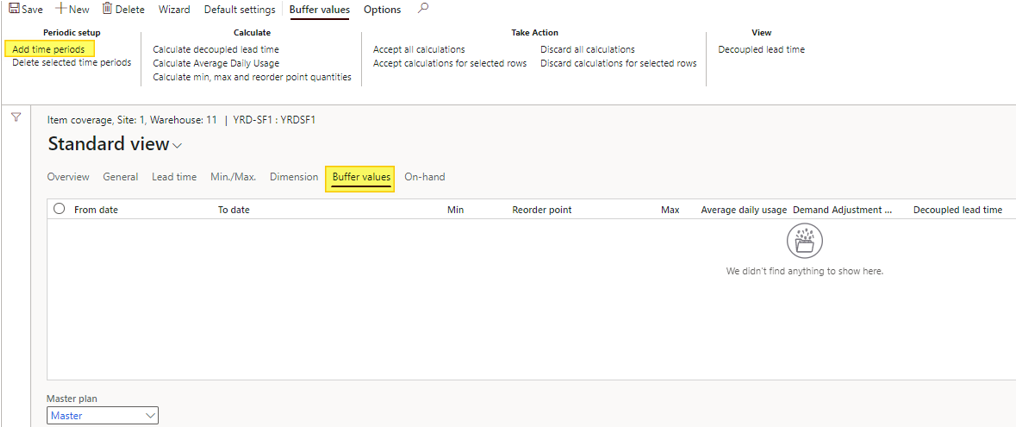Buffer lines are added depending on the frequency (daily means one line per day, weekly means one line per week).

The frequency is setup under the “Min, max and reorder-point period” and the number of lines generated depends also of the “Coverage time fence (days)”.For instance, coverage time fence of 5 days with a weekly basis will generate only one buffer line. On a Daily basis it would have generated 5 lines.

Let’s see a result example: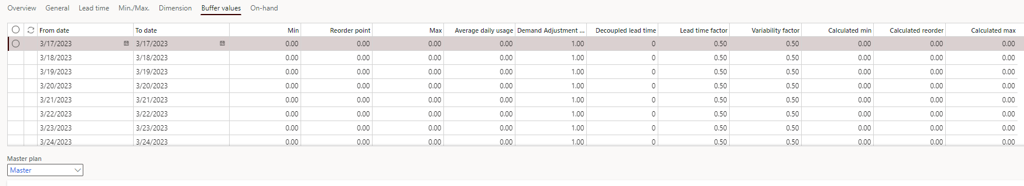Then, you have to run the calculation : Clic on Calculate decoupled lead time, then Calculate Average Daily Usage and finaly Calculate min, max and reorder point quantities .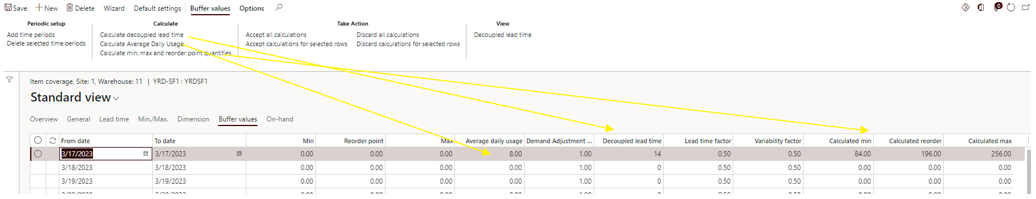Under the Buffer value tab, it’s possible to Accept all calculations.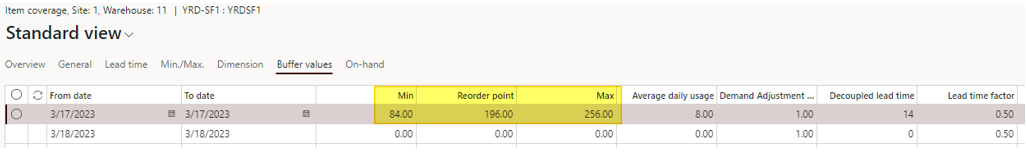Then, the Min, Reorder point and Max values have been initialized, for each period if you have data. It’s always possible to override the calculated values.

## With more data

Adding more data helps you analyse the stock view across days or weeks, as you can see in the screenshot below.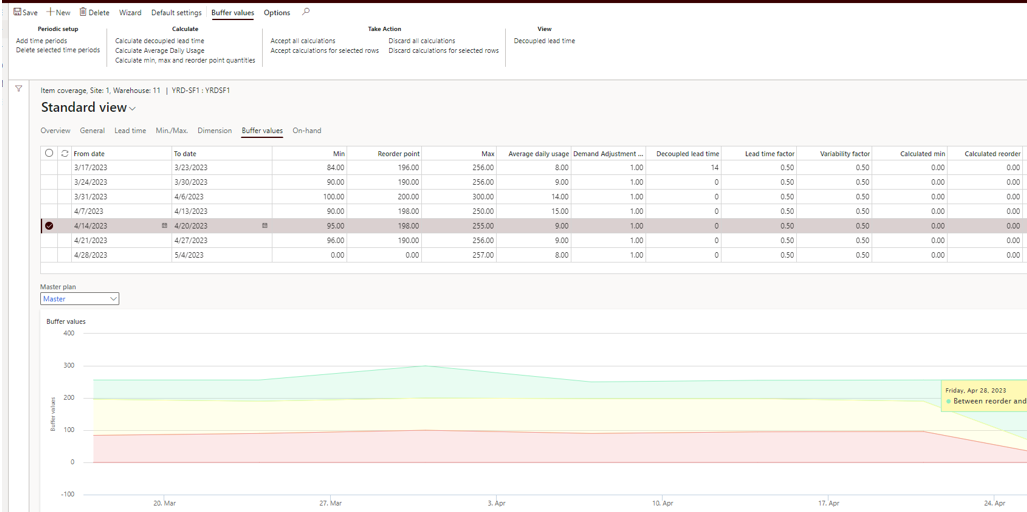Note that you can delete lines by selecting lines and choosing the Delete selected time periods or through ALT + F9.

Another interesting feature is the possibility to adjust the proposal before accepting them. For instance if you know for seasonality reasons that the demand will be far much higher than calculated, you can use the Demand Adjustment Factor to weight any line.

In the example below, I’ve put a rate of 5, and run the calculation again.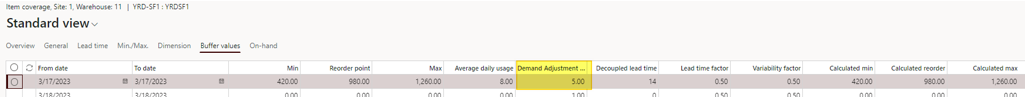As you can see, the Calculated min, Calculated reorder and Calculated max have been updated accordingly. Note that you can adjust only if Buffer value over time is setup to yes.

## Automatization

Go under Master planning > Master planning > DDMRP > Calculate buffer values for automatize and batch those calculations.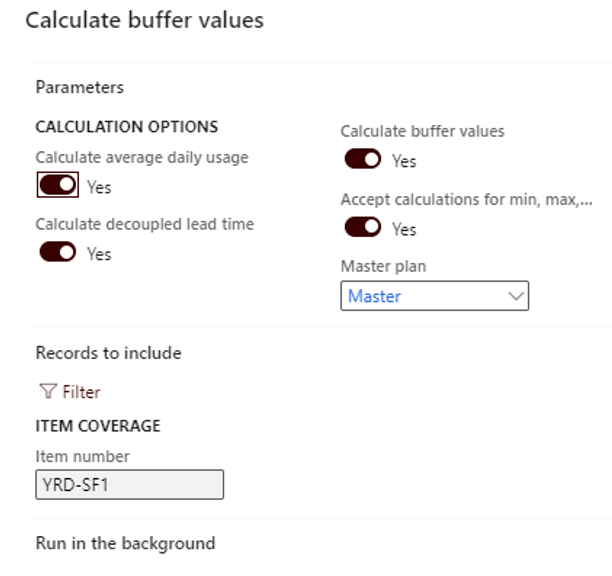Retrieve same result

You will retrieve the same result as done manually.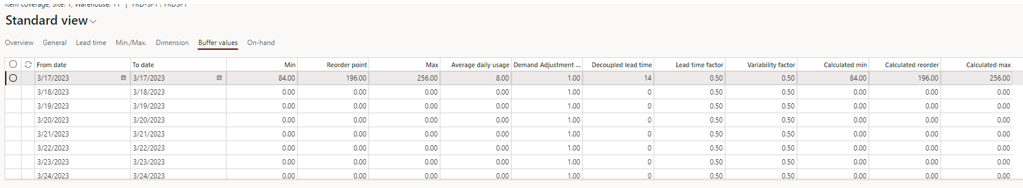In the next post, we will see how the DDMRP is run and analyses the results for planned orders.

Yohann

Ce site utilise Akismet pour réduire les indésirables. En savoir plus sur comment les données de vos commentaires sont utilisées.